Electron. J. Diff. Equ., Vol. 2010(2010), No. 27, pp. 1-7.

### Exact multiplicity of solutions for a class of two-point boundary value problems Yulian An, Ruyun Ma

Abstract:
We consider the exact multiplicity of nodal solutions of the boundary value problem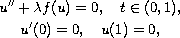where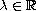is a positive parameter.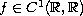satisfies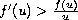, if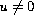. There exist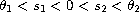such that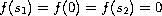;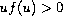, if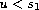or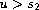;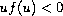, if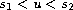and;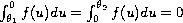. The limit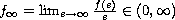. Using bifurcation techniques and the Sturm comparison theorem, we obtain curves of solutions which bifurcate from infinity at the eigenvalues of the corresponding linear problem, and obtain the exact multiplicity of solutions to the problem forlying in some interval in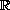.

Submitted September 30, 2009. Published February 16, 2010.
Math Subject Classifications: 34B15, 34A23.
Key Words: Exact multiplicity; nodal solutions; bifurcation from infinity; linear eigenvalue problem.

Show me the PDF file (222 KB), TEX file, and other files for this article.Yulian An Department of Mathematics Shanghai Institute of Technology shanghai 200235, China email: an_yulian@tom.comRuyun Ma Department of Mathematics Northwest Normal University Lanzhou 730070, Gansu, China email: mary@nwnu.edu.cn